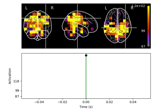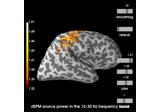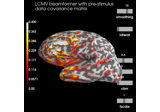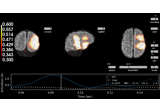# mne.beamformer.make_lcmv¶

mne.beamformer.make_lcmv(info, forward, data_cov, reg=0.05, noise_cov=None, label=None, pick_ori=None, rank='info', weight_norm='unit-noise-gain-invariant', reduce_rank=False, depth=None, inversion='matrix', verbose=None)[source]

Compute LCMV spatial filter.

Parameters
infoinstance of Info

The measurement info to specify the channels to include. Bad channels in info[‘bads’] are not used.

forwardinstance of Forward

Forward operator.

data_covinstance of Covariance

The data covariance.

regfloat

The regularization for the whitened data covariance.

noise_covinstance of Covariance

The noise covariance. If provided, whitening will be done. Providing a noise covariance is mandatory if you mix sensor types, e.g. gradiometers with magnetometers or EEG with MEG.

labelinstance of Label

Restricts the LCMV solution to a given label.

pick_ori

For forward solutions with fixed orientation, None (default) must be used and a scalar beamformer is computed. For free-orientation forward solutions, a vector beamformer is computed and:

• None

Orientations are pooled after computing a vector beamformer (Default).

• 'normal'

Filters are computed for the orientation tangential to the cortical surface.

• 'max-power'

Filters are computed for the orientation that maximizes power.

• 'vector'

Keeps the currents for each direction separate

rankNone | dict | ‘info’ | ‘full’

This controls the rank computation that can be read from the measurement info or estimated from the data. See Notes of mne.compute_rank() for details.The default is “info”.

weight_norm

Can be:

• None

The unit-gain LCMV beamformer 1 will be computed.

• 'unit-noise-gain'

The unit-noise gain minimum variance beamformer will be computed (Borgiotti-Kaplan beamformer) 1, which is not rotation invariant when pick_ori='vector'. This should be combined with stc.project('pca') to follow the definition in 1.

• 'nai'

The Neural Activity Index 2 will be computed, which simply scales all values from 'unit-noise-gain' by a fixed value.

• 'unit-noise-gain-invariante'

Compute a rotation-invariant normalization using the matrix square root. This differs from 'unit-noise-gain' only when pick_ori='vector', creating a solution that:

1. Is rotation invariant ('unit-noise-gain' is not);

2. Satisfies the first requirement from 1 that w @ w.conj().T == I, whereas 'unit-noise-gain' has non-zero off-diagonals; but

3. Does not satisfy the second requirement that w @ G.T = θI, which arguably does not make sense for a rotation-invariant solution.

Defaults to 'unit-noise-gain-invariant'.

reduce_rankbool

If True, the rank of the denominator of the beamformer formula (i.e., during pseudo-inversion) will be reduced by one for each spatial location. Setting reduce_rank=True is typically necessary if you use a single sphere model with MEG data.

Changed in version 0.20: Support for reducing rank in all modes (previously only supported pick='max_power' with weight normalization).

depth

How to weight (or normalize) the forward using a depth prior. If float (default 0.8), it acts as the depth weighting exponent (exp) to use, which must be between 0 and 1. None is equivalent to 0, meaning no depth weighting is performed. It can also be a dict containing keyword arguments to pass to mne.forward.compute_depth_prior() (see docstring for details and defaults). This is effectively ignored when method='eLORETA'.

Changed in version 0.20: Depth bias ignored for method='eLORETA'.

New in version 0.18.

inversion‘single’ | ‘matrix’

This determines how the beamformer deals with source spaces in “free” orientation. Such source spaces define three orthogonal dipoles at each source point. When inversion='single', each dipole is considered as an individual source and the corresponding spatial filter is computed for each dipole separately. When inversion='matrix', all three dipoles at a source vertex are considered as a group and the spatial filters are computed jointly using a matrix inversion. While inversion='single' is more stable, inversion='matrix' is more precise. See section 5 of 3. Defaults to 'matrix'.

New in version 0.21.

verbose

If not None, override default verbose level (see mne.verbose() and Logging documentation for more). If used, it should be passed as a keyword-argument only.

Returns
filtersinstance of Beamformer

Dictionary containing filter weights from LCMV beamformer. Contains the following keys:

‘kind’str

The type of beamformer, in this case ‘LCMV’.

‘weights’array

The filter weights of the beamformer.

‘data_cov’instance of Covariance

The data covariance matrix used to compute the beamformer.

‘noise_cov’instance of Covariance | None

The noise covariance matrix used to compute the beamformer.

‘whitener’None | ndarray, shape (n_channels, n_channels)

Whitening matrix, provided if whitening was applied to the covariance matrix and leadfield during computation of the beamformer weights.

‘weight_norm’str | None

Type of weight normalization used to compute the filter weights.

‘pick-ori’None | ‘max-power’ | ‘normal’ | ‘vector’

The orientation in which the beamformer filters were computed.

‘ch_names’list of str

Channels used to compute the beamformer.

‘proj’array

Projections used to compute the beamformer.

‘is_ssp’bool

If True, projections were applied prior to filter computation.

‘vertices’list

Vertices for which the filter weights were computed.

‘is_free_ori’bool

If True, the filter was computed with free source orientation.

‘n_sources’int

Number of source location for which the filter weight were computed.

‘src_type’str

Type of source space.

‘source_nn’ndarray, shape (n_sources, 3)

For each source location, the surface normal.

‘proj’ndarray, shape (n_channels, n_channels)

Projections used to compute the beamformer.

‘subject’str

The subject ID.

‘rank’int

The rank of the data covariance matrix used to compute the beamformer weights.

‘max-power-ori’ndarray, shape (n_sources, 3) | None

When pick_ori=’max-power’, this fields contains the estimated direction of maximum power at each source location.

‘inversion’‘single’ | ‘matrix’

Whether the spatial filters were computed for each dipole separately or jointly for all dipoles at each vertex using a matrix inversion.

Notes

The original reference is 2.

To obtain the Sekihara unit-noise-gain vector beamformer, you should use weight_norm='unit-noise-gain', pick_ori='vector' followed by vec_stc.project('pca', src).

Changed in version 0.21: The computations were extensively reworked, and the default for weight_norm was set to 'unit-noise-gain-invariant'.

References

1(1,2,3,4)

Kensuke Sekihara and Srikantan S. Nagarajan. Adaptive Spatial Filters for Electromagnetic Brain Imaging. Series in Biomedical Engineering. Springer, Berlin; Heidelberg, 2008. ISBN 978-3-540-79369-4 978-3-540-79370-0. doi:10.1007/978-3-540-79370-0.

2(1,2)

Barry D. Van Veen, Wim van Drongelen, Moshe Yuchtman, and Akifumi Suzuki. Localization of brain electrical activity via linearly constrained minimum variance spatial filtering. IEEE Transactions on Biomedical Engineering, 44(9):867–880, 1997. doi:10.1109/10.623056.

3

Marijn van Vliet, Mia Liljeström, Susanna Aro, Riitta Salmelin, and Jan Kujala. Analysis of functional connectivity and oscillatory power using DICS: from raw MEG data to group-level statistics in Python. bioRxiv, 2018. doi:10.1101/245530.

## Examples using mne.beamformer.make_lcmv¶